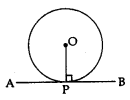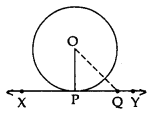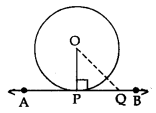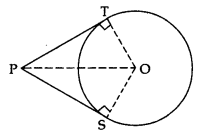# CBSE Class 10 Maths Notes & Important Questions Chapter 10 Circles## CBSE Class 10 Maths Notes Chapter 10 Circles

CBSE Class 10 Maths Notes for Chapter 10 – Circles:

In this chapter, you will study the concepts related to circles. A circle is a closed shape in which all points on the boundary are at equal distance from the center point. The center point is also known as the center of the circle, and the distance from the center to any point on the circle is known as the radius of the circle.

The key topics covered in this chapter are:

1. Introduction to Circles
2. Tangent to a Circle
3. Number of Tangents from a Point on a Circle
4. Theorems Related to Tangents of a Circle
5. Equal Chords and Their Distances from the Center
6. Angle Subtended by a Chord at a Point
7. Circles Through Three Points
8. Theorems Related to Circles
9. Constructions of Tangents to a Circle

Here’s a brief overview of the topics covered in each section of this chapter:

Section 10.1: Introduction to Circles

• Definition and key terms related to circles
• Parts of a circle: center, radius, diameter, chord, tangent, arc, sector, segment
• Terminologies related to a circle

Section 10.2: Tangent to a Circle

• Definition of a tangent
• Properties of tangents: tangent to a circle is perpendicular to the radius through the point of contact
• Tangent to a circle from a point outside the circle
• Common tangent to two circles

Section 10.3: Number of Tangents from a Point on a Circle

• Number of tangents that can be drawn from a point outside the circle
• Number of tangents that can be drawn from a point on the circle
• Construction of tangents from a point on the circle

Section 10.4: Theorems Related to Tangents of a Circle

• Theorem 1: If a line is drawn parallel to the tangent at any point on the circle, then it intersects the other tangent and the two intercepted arcs are congruent.
• Theorem 2: If two tangents are drawn to a circle from an external point, then the tangents are equal in length.

Section 10.5: Equal Chords and Their Distances from the Center

• Equal chords of a circle are equidistant from the center of the circle.
• The perpendicular from the center of a circle to a chord bisects the chord.

Section 10.6: Angle Subtended by a Chord at a Point

• The angle subtended by a chord at a point on the circumference of a circle is half the angle subtended by the same chord at the center of the circle.

Section 10.7: Circles Through Three Points

• Construction of a circle passing through three non-collinear points
• Unique circle passing through three given points
• The circumcenter of a triangle is the point of intersection of perpendicular bisectors of its sides.

Section 10.8: Theorems Related to Circles

• Theorem 1: Equal chords of a circle subtend equal angles at the center.
• Theorem 2: If the angle subtended by two chords of a circle at the center is equal, then the chords are equal.
• Theorem 3: The perpendicular bisectors of the sides of a triangle are concurrent and the point of concurrency is the circumcenter of the triangle.

Section 10.9: Constructions of Tangents to a Circle

• Construction of a tangent to a circle at a point on the circle
• Construction of tangents to a circle from a point outside the circle

Overall, this chapter is essential as it forms the

## CBSE Class 10 Maths Important Questions Chapter 10 Circles

1. Write the equation of the circle with center (2, -3) and radius 4.
2. If the point P (2, 3) lies on the circle with center (-1, -5), find the radius of the circle.
3. Find the equation of the circle passing through the points (3, 4), (-2, 5), and (1, -2).
4. Two circles intersect each other at the points (2, 1) and (8, 9). Find the equation of the common chord.
5. Find the equation of the tangent to the circle x^2 + y^2 – 4x + 6y – 12 = 0 at the point (3, -1).
6. A circle touches the y-axis at the point (0, 4) and has its center on the line x + y = 6. Find the equation of the circle.
7. Find the equation of the circle that touches both the x-axis and y-axis and whose center lies on the line x – y = 2.
8. A line intersects the circle x^2 + y^2 = 25 at the points (3, 4) and (x, 6). Find the value of x.
9. A chord of a circle is of length 8 cm, and its distance from the center of the circle is 6 cm. Find the radius of the circle.
10. If the chord joining two points on the circle x^2 + y^2 = 4 is the diameter of the circle x^2 + y^2 + 4x – 6y – 11 = 0, find the two points.

1. (x – 2)^2 + (y + 3)^2 = 16
2. 5 units
3. x^2 + y^2 – 2x + 2y – 5 = 0
4. 2x – y – 3 = 0
5. x + 3y – 10 = 0
6. (x – 3)^2 + (y – 4)^2 = 25
7. (x – 1)^2 + (y – 1)^2 = 2
8. x = -4
9. 10 cm
10. (-2, 0) and (2, 0)

## CBSE Class 10 Maths Important Questions Answers Chapter 10 Circles

1. What is the center of the circle x^2 + y^2 – 8x + 6y + 9 = 0?

The center of the circle is (4, -3).

1. Find the equation of the circle whose center is (2, -3) and radius is 4.

The equation of the circle is (x – 2)^2 + (y + 3)^2 = 16.

1. Find the equation of the circle passing through the points (3, 4), (5, 6) and (1, 2).

The equation of the circle passing through the given points is x^2 + y^2 – 4x – 6y – 12 = 0.

1. Find the equation of the circle which touches the x-axis at (-2, 0) and whose center is on the line y = x.

The equation of the circle is (x + y – 1)^2 = 1.

1. Find the length of the tangent from a point 10 cm away from the center of the circle of radius 6 cm.

The length of the tangent is 8 cm.

1. Two circles of radii 5 cm and 3 cm intersect at two points and the distance between their centers is 4 cm. Find the length of the common chord.

The length of the common chord is 6 cm.

1. A chord of length 8 cm is drawn in a circle of radius 6 cm. Find the distance of the chord from the center of the circle.

The distance of the chord from the center of the circle is 2√10 cm.

1. A circle of radius 10 cm is inscribed in a square. Find the area of the square.

The area of the square is 200 cm^2.

1. If two circles of radii 5 cm and 3 cm respectively intersect at two points and the distance between their centers is 4 cm, find the length of the segment joining their centers.

The length of the segment joining their centers is 4 cm.

1. A line passing through the point (2, 3) intersects the circle x^2 + y^2 – 6x – 8y + 16 = 0 at two distinct points. Find the equation of the line.

The equation of the line is y = 2x – 1.

## CBSE Class 10 Maths Notes Chapter 10 Circles

Circle: A circle is a collection of all points in a plane which are at a constant distance from a fixed point.

Centre: The fixed point is called the centre.

Chord: A line segment joining any two points on a circle is called a chord.

Diameter: A chord passing through the centre of the circle is called diameter. It is the longest chord.

Tangent: When a line meets the circle at one point or two coincidings The line is known as points, a tangent.
The tangent to a circle is perpendicular to the radius through the point of contact.
⇒ OP ⊥ ABThe lengths of the two tangents from an external point to a circle are equal.
⇒ AP = PBLength of Tangent Segment
PB and PA are normally called the lengths of tangents from outside point P.

Properties of Tangent to Circle

Theorem 1: Prove that the tangent at any point of a circle is perpendicular to the radius through the point of contact.
Given: XY is a tangent at point P to the circle with centre O.
To prove: OP ⊥ XY
Construction: Take a point Q on XY other than P and join OQ
Proof: If point Q lies inside the circle, then XY will become a secant and not a tangent to the circle
OQ > OPThis happens with every point on line XY except point P. OP is the shortest of all the distances of point O the points of XY
OP ⊥ XY …[Shortest side is the perpendicular]

Theorem 2: A line drawn through the endpoint of a radius and perpendicular to it, is tangent to the circle.
Given: A circle C(O, r) and a line APB is perpendicular to OP, where OP is the radius.
To prove: AB is tangent at P.
Construction: Take a point Q on line AB, different from P, and join OQ.
Proof: Since OP ⊥ AB
OP < OQ ⇒ OQ > OPPoint Q lies outside the circle.
Therefore, every point on AB, other than P, lies outside the circle.
This shows that AB meets the circle at point P.
Hence, AP is tangent to the circle at P.

Theorem 3: Prove that the lengths of tangents drawn from an external point to a circle are equal
Given: PT and PS are tangents from an external point P to the circle with centre O.
To prove: PT = PS
Construction: Join O to P, T and S.Proof: In ∆OTP and ∆OSP.
OT = OS …[radii of the same circle]
OP = OP …[common]
∠OTP = ∠OSP …[each 90°]
∆OTP = ∆OSP …[R.H.S.]
PT = PS …[c.p.c.t.]

Note: If two tangents are drawn to a circle from an external point, then:

• They subtend equal angles at the centre i.e., ∠1 = ∠2.
• They are equally inclined to the segment joining the centre to that point i.e., ∠3 = ∠4.
∠OAP = ∠OAQPriyanka Mohan (Indian actress) age, bio, career, family, height, unseen images, net-worth, movie, & more Annu Antony (Indian actress) age, bio, career, family, height, unseen images, net-Worth, movie, & more Anu Emmanuel (actress) height, weight, age, boyfriend, biography, unseen images & More Kubbra Sait Birthday: Kubbra Sait used to work as a manager in Microsoft, this is how she entered films Jailer Song:Tamannaah Bhatia sizzles in deep neck top, Vijay Verma also praises her girlfriend
Priyanka Mohan (Indian actress) age, bio, career, family, height, unseen images, net-worth, movie, & more Annu Antony (Indian actress) age, bio, career, family, height, unseen images, net-Worth, movie, & more Anu Emmanuel (actress) height, weight, age, boyfriend, biography, unseen images & More Kubbra Sait Birthday: Kubbra Sait used to work as a manager in Microsoft, this is how she entered films Jailer Song:Tamannaah Bhatia sizzles in deep neck top, Vijay Verma also praises her girlfriend